# 中山市东菱照明科技有限公司上线成功

2020-11-03 11:13CRM客户管理：客户档案管理（客户基础资料、决策链条、主销/在研项目、主材供应等）、项目管理（大客户项目、新产品项目、样品项目）、商机管理、工作任务（销售任务、出差记录、打卡记录）、产品阶梯价格管理、竞争管理（竞争对手、友商大客户、立项分析）等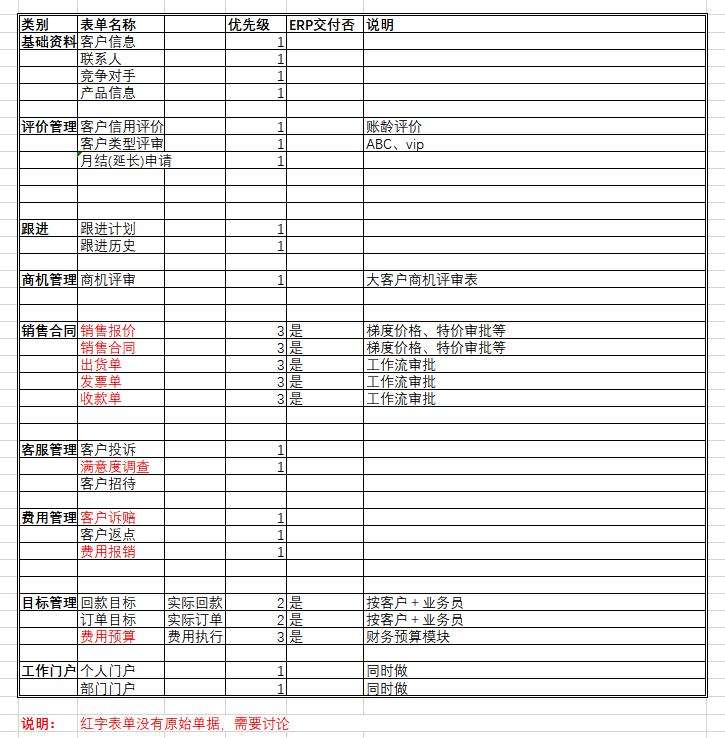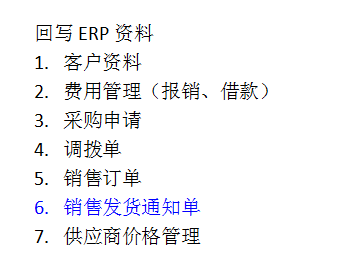、应用解读

2.1 CRM客户管理：CRM客户管理是公司的核心业务系统，目的是以项目管理模式推动销售管理升级，以下从业务核心要素、实施要点、销售逻辑、信息化管理流程来介绍CRM客户管理的实施成果。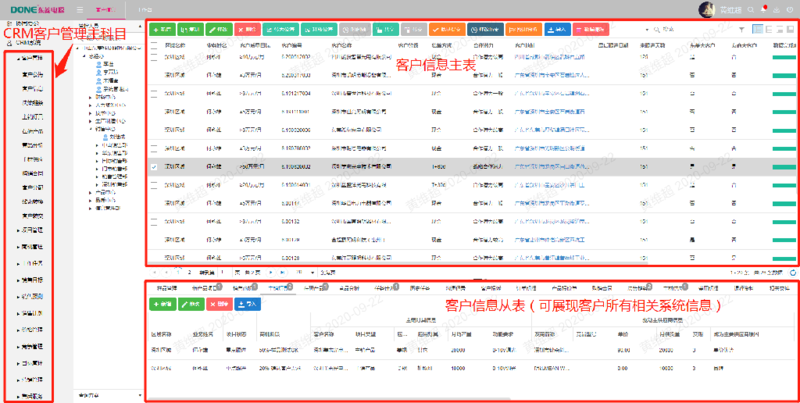2.1.1 CRM管理的核心要素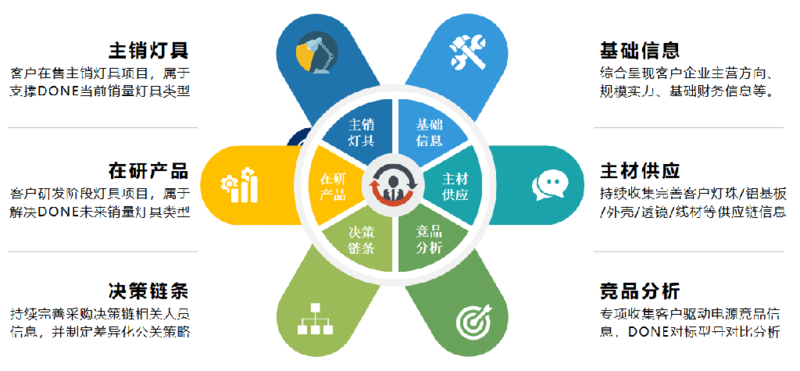CRM的核心包括客户基础信息、决策链条、主材供应、主销灯具、在研产品、竞品，其中客户信息是需要通过新客户报备审批流程来管控并且同步到ERP系统。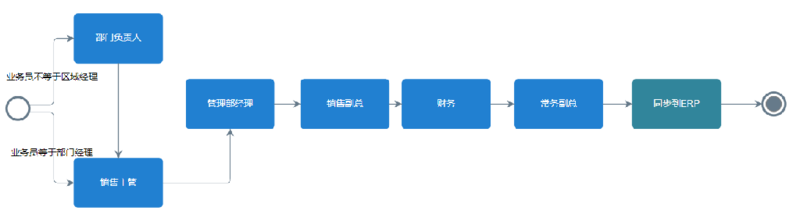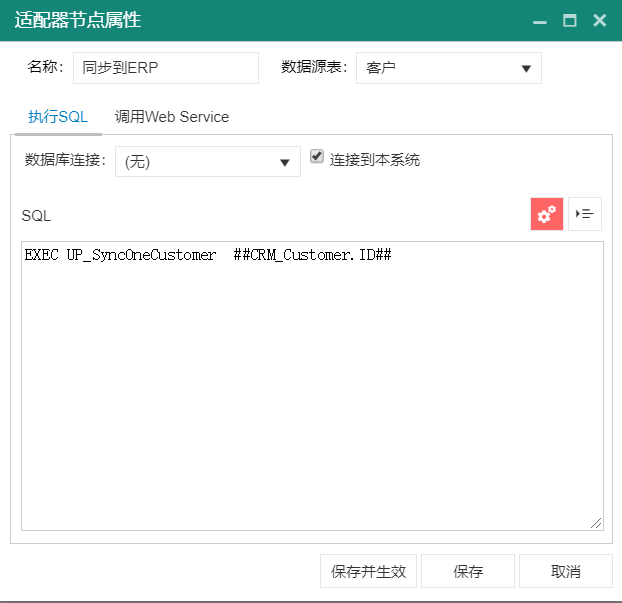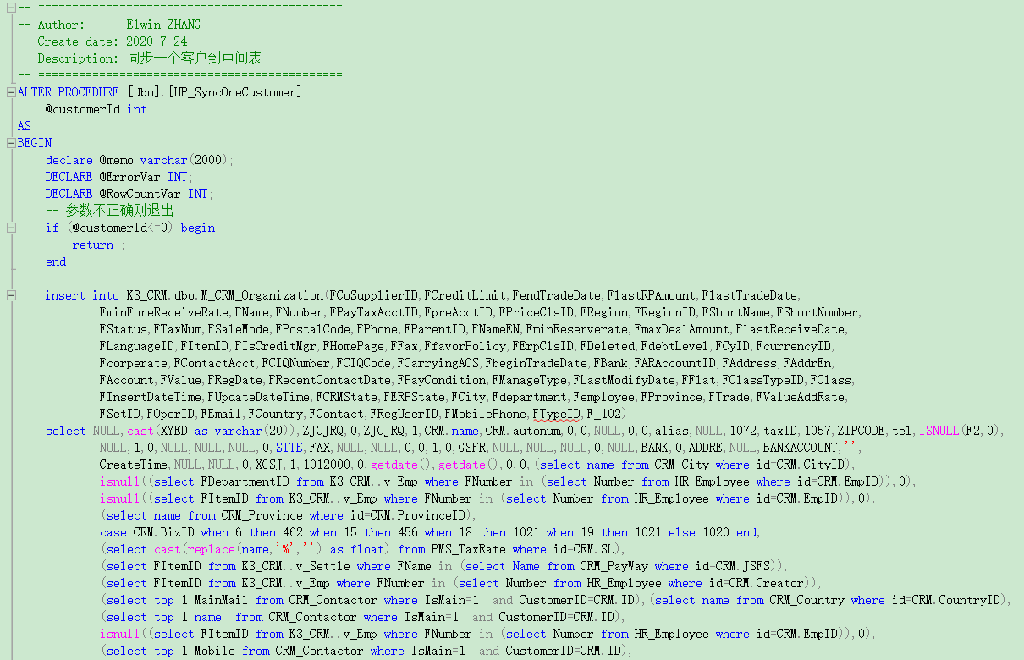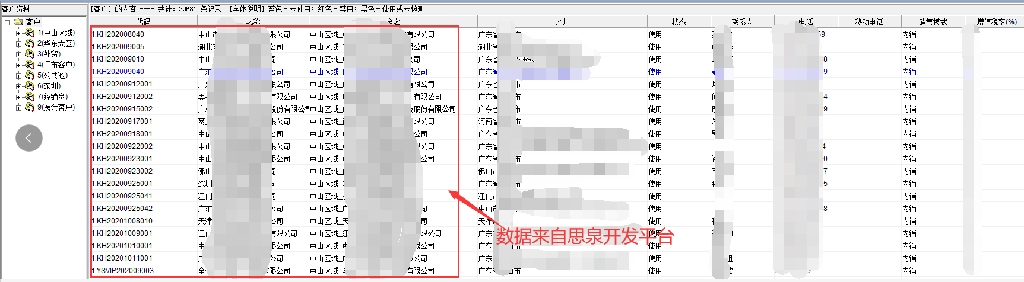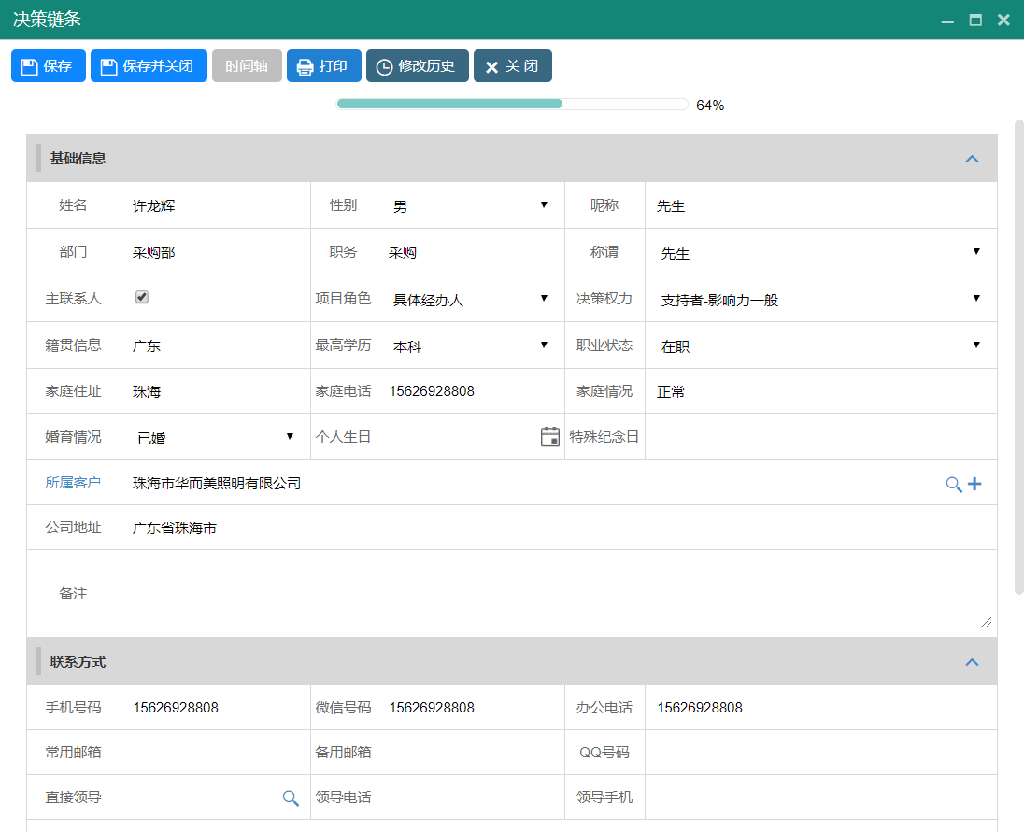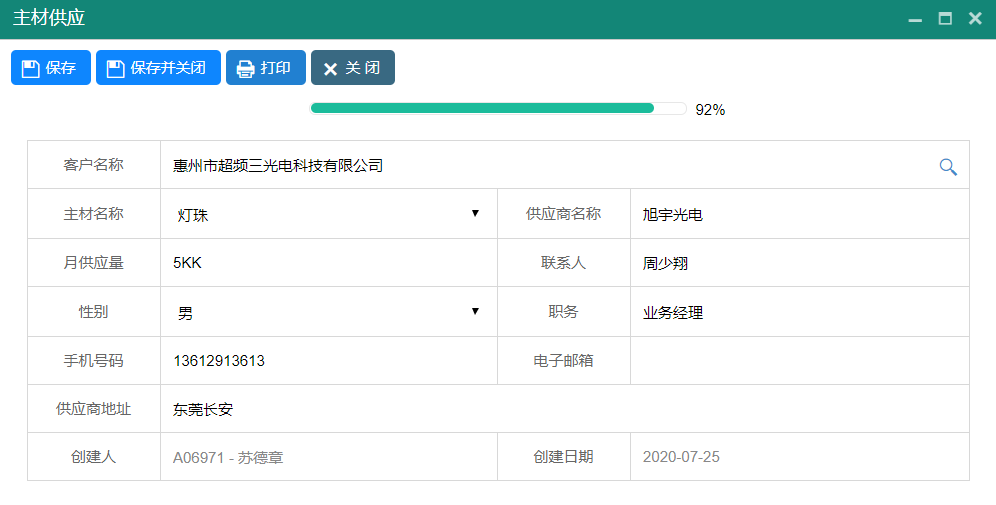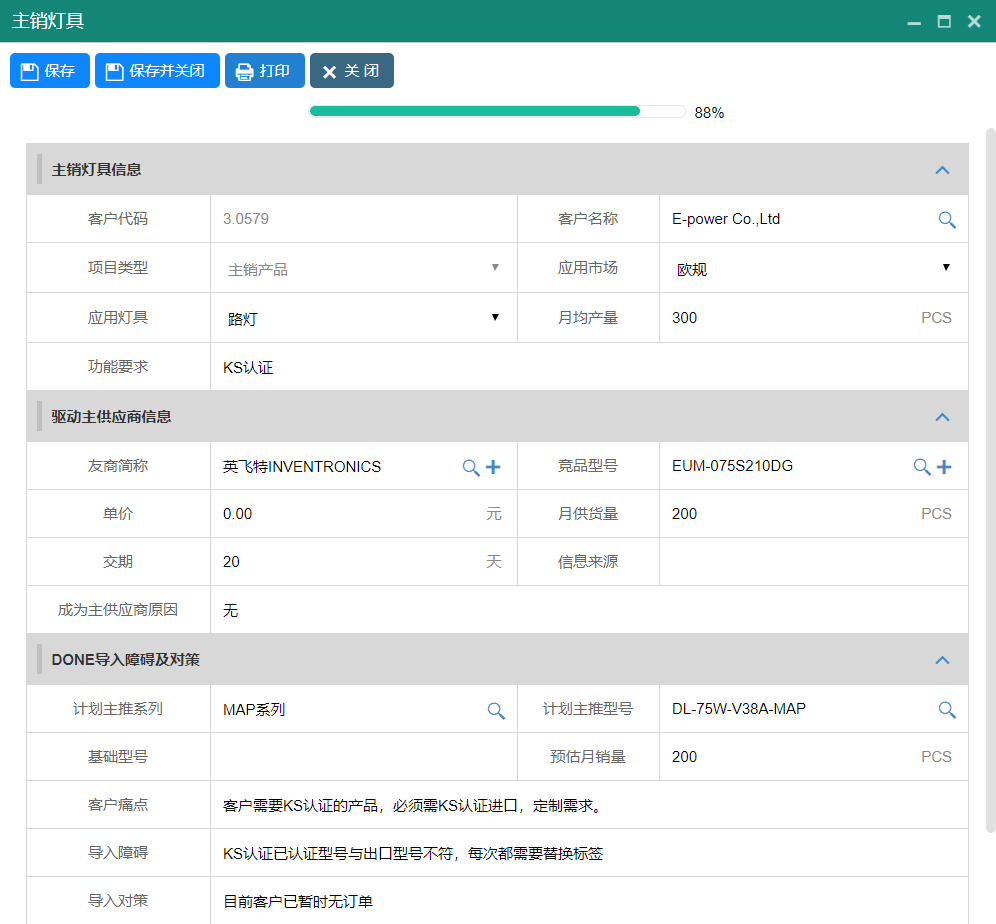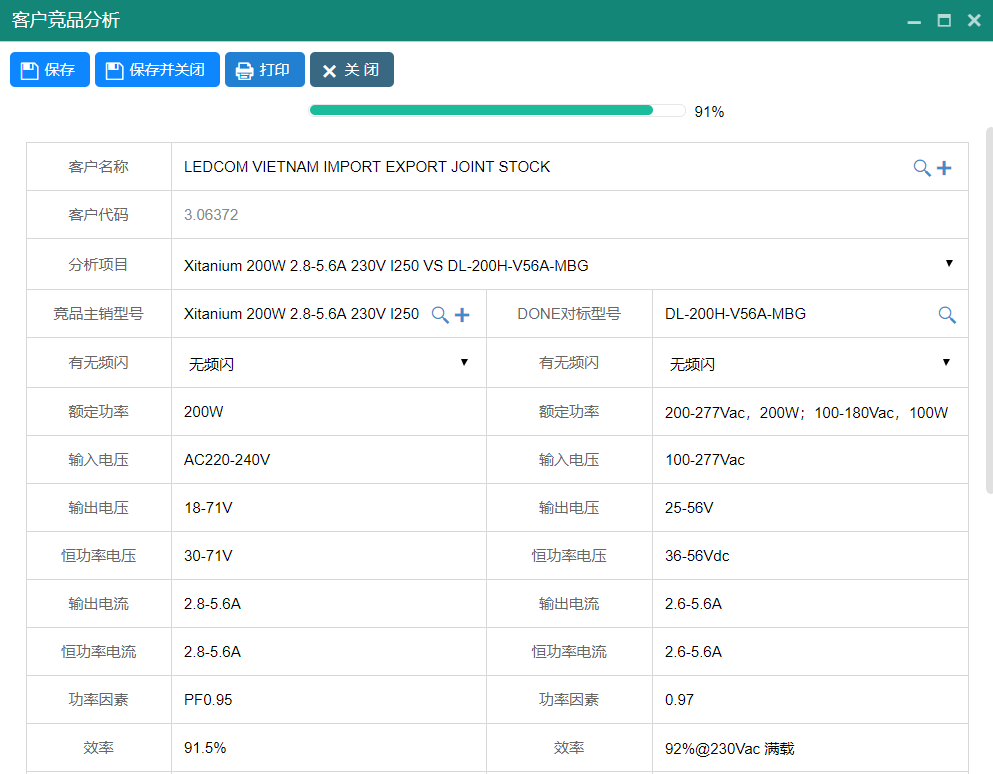2.1.2 CRM客户管理实施要点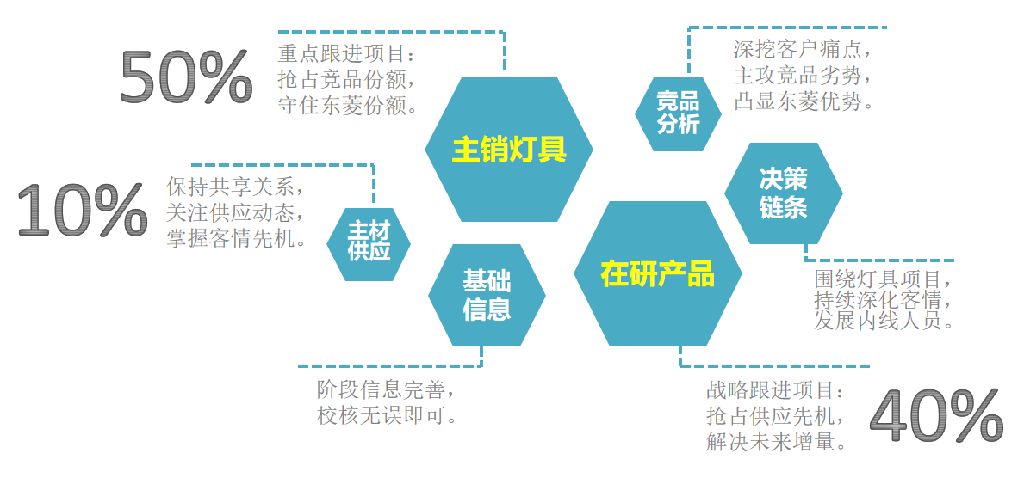2.1.3 围绕客户项目开展的销售逻辑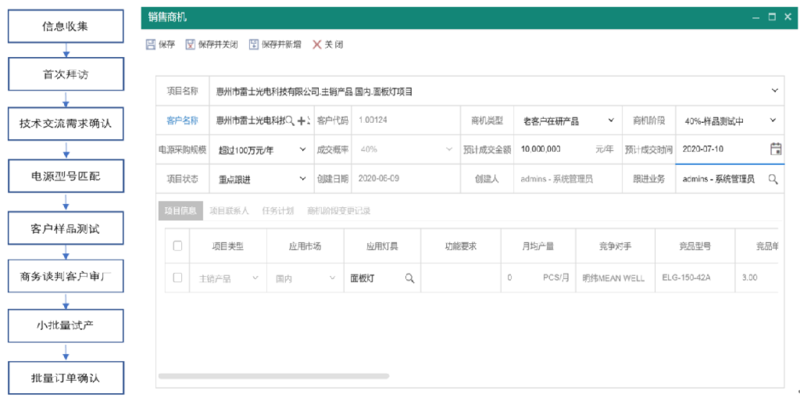2.1.4 销售业务的信息化管理2.1.5 CRM上线模块2.2 产品管理

2.2.1 产品信息是业务系统的核心基础资料。2.2.2 价格管理：产品阶梯价、促销价2.3 OA协同办公//数量改变后触发获取参考价格事件function getProdPrice() {    var qty = \$(this).val();   //数量    if (qty == null || qty == '') {        qty = 0;    } else {        qty = parseInt(qty.replace(/,/g, ''));    }    var custId = \$('#KHMC').attr('idvalue');    if (custId == null || custId == '') {        return;    }    var tr = \$(this).closest('tr');    var prodId = \$(tr).find('[sname="CPMC"]').attr('idvalue');    if (prodId == null || prodId == '') {        return;    }    var sql = 'EXEC [UP_GET_PROD_PRICE] ' + prodId + ',' + custId + ',' + qty;    var url = '../siquan/Access.ashx';    \$.post(url, { action: 'getvalue', sql: sql }, function (data) {      debugger;        \$(tr).find('[sname="DBPrice"]').val(data);        \$(tr).find('[sname="HSDJ"]').val(data);        \$(tr).find('[sname="HJDJ"]').val(data);        calculateMoney(tr);    });}//修改含税单价事件function changeHSDJ() {    var tr = \$(this).closest('tr');    calculateMoney(tr);}//选择产品之后触发事件function afterChooseProd(prodId, textValue, ctl) {   　debugger;    var tr = \$(ctl).closest('tr');    //清空后面的数量价格金额    \$(tr).find('[sname="SL"]').val('');    \$(tr).find('[sname="DBPrice"]').val('');    \$(tr).find('[sname="HSDJ"]').val('');    \$(tr).find('[sname="XJ"]').val('');    //经理价    var sql = 'select JG from CRM_JTJ where CPMC=' + prodId + ' and JTJMC=2';    var url = '../siquan/Access.ashx';    \$.post(url, { action: 'getvalue', sql: sql }, function (data) {        \$(tr).find('[sname="DYJLJ"]').attr('vvv', data);    });    //副总价    sql = 'select JG from CRM_JTJ where CPMC=' + prodId + ' and JTJMC=3';    \$.post(url, { action: 'getvalue', sql: sql }, function (data) {        \$(tr).find('[sname="DYFZJ"]').attr('vvv', data);    });}//重新计算金额,比较价格function calculateMoney(tr) {    var qty = \$(tr).find('[sname="SL"]').val();    if (qty == null || qty == '') {        qty = 0;    } else {        qty = parseInt(qty.replace(/,/g, ''));    }    var dj1 = \$(tr).find('[sname="HSDJ"]').val();    if (dj1 == null || dj1 == '') {        dj1 = 0;    } else {        dj1 = parseFloat(dj1.replace(/,/g, ''));    }    var vvv = "2";    //经理价 2       var jlj = \$(tr).find('[sname="DYJLJ"]').attr('vvv');    if (dj1 < jlj) { vvv = "1" };    \$(tr).find('[sname="DYJLJ"]').val(vvv);    vvv = "2";    //副总价 3       var fzj = \$(tr).find('[sname="DYFZJ"]').attr('vvv');    if (dj1 < fzj) { vvv = "1" };    \$(tr).find('[sname="DYFZJ"]').val(vvv);    var dj2 = \$(tr).find('[sname="XPDJ"]').val();    if (dj2 == null   || dj2 == '') {        dj2 = 0;    } else {        dj2 = parseFloat(dj2.replace(/,/g, ''));    }    \$(tr).find('[sname="XJ"]').val(qty * (dj1 + dj2));}LED驱动器元器件材料清单：10/27

2023

09/26

2023

06/25

2022

03/24

2021

02/18

2021

深圳华云信息系统有限公司(http://www.huayunsoft.com)成立于2007年05月14日，注册地位于深圳市南山区粤海街道高新南七道数字技术园国家工程实验室大楼A座904号。经营范围包括一般经营项目是：人工智能及大数...

11/03

2020

中山市东菱照明科技有限公司创立于2009年，其前身为中山市古镇菱菱电子厂，一路风雨、一路成长，公司经过七年多的飞跃发展，于2015年喜迁至享有“中国照明灯饰

 工作时间周一至周六 ：8:30-18:00 联系方式客服热线：4000-1189-81总部电话：0755-86030152企业微信